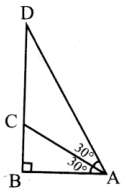Tamilnadu State Board New Syllabus Samacheer Kalvi 8th Maths Guide Pdf Chapter 5 Geometry Ex 5.1 Text Book Back Questions and Answers, Notes.

## Tamilnadu Samacheer Kalvi 8th Maths Solutions Chapter 5 Geometry Ex 5.1

Question 1.
Fill in the blanks with the correct term from the given list.
(in proportion, similar, corresponding, congruent, shape, area, equal)
(i) Corresponding sides of similar triangles are _________ .
in proportion

(ii) Similar triangles have the same ________ but not necessarily the same size.
Shape(iii) In any triangle _______ sides are opposite to equal angles.
equal

(iv) The symbol is used to represent _________ triangles.
congruent

(v) The symbol ~ is used to represent _________ triangles.
similar

Question 2.
In the figure, ∠CIP ≡ ∠COP and ∠HIP ≡ ∠HOP. Prove that IP ≡ OP.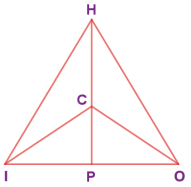Statements Reasons 1. CI = CO ∵ CIP ≡ COP, by CPCTC 2. IP =  OP By CPCTC 3. CP = CP By CPCTC 4. Also HI = HO CPCTC ∆HIP ≡ HOP given 5. IP = OP By CPCTC and (4) 6. ∴ IP ≡ OP By (2) and (4)Question 3.
In the given figure, AC ≡ AD and ∠CBD ≡ ∠DEC. Prove that ∆ BCF ≡ ∆ EDF.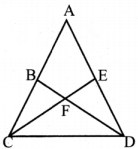Statements Reasons 1. ∠BCF = ∠EFD Vertically opposite angles 2. ∠CBD = ∠DEC Angles on the same base given 3. ∠BCF = ∠EDF Remaining angles of ∆BCF and ∆EDF 4. ∆BCF ≡ ∆EDF By (1) and (2) AAA criteriaQuestion 4.
In the given figure, △ BCD is isosceles with base BD and ∠BAE ≡∠DEA. Prove that AB ≡ ED.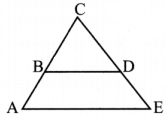Statements Reasons 1. ∠BAE ≡ ∠DEA Given 2. AC = EC By (1) sides opposite to equal angles are equal 3. BC = DC Given BCD is isosceles with base BD 4. AC – BC = EC – DC 2 – 3 5. AB ≡ ED By 4

Question 5.
In the given figure, D is the midpoint of OE and ∠CDE = 90°. Prove that △ODC ≡ △EDC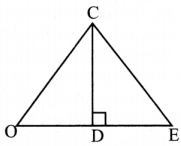Statements Reasons 1. OD = ED D is the midpoint OE (given) 2. DC = DC Common side 3. ∠CDE = ∠CDO = 90° Linear pair and given ∠CDE = 90° 4. △ODC ≡ △EDC By RHS criteriaQuestion 6.
Is △PRQ ≡ △QSP? Why?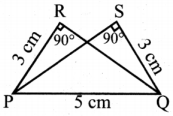In △PRQ and △PSQ
∠PRQ = ∠PSQ = 90° given
PR = QS = 3 cm given
PQ = PQ = 5 cm common
It satisfies RHS criteria
∴ △PRQ congruent to △QSP.Question 7.
From the given figure, prove that △ABC ~ △EDF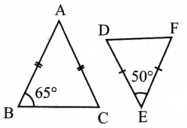From the △ABC, AB = AC
It is an isosceles triangle
Angles opposite to equal sides are equal
∴ ∠B = ∠C = 65°
∴ ∠B + ∠C = 65° + 65°
= 130°
We know that sum of three angles is a triangle = 180°
∠A + ∠B + ∠C = 180°
∠A + 130° = 180°
∠A = 180° – 130°
∠A = 50°
From △EDF, ∠E = 50°
∴ Sum of Remaining angles = 180° – 50° = 130°
DE = FD
∴ ∠D = ∠F
From △ABC and △EDF ∴ △D = $$\frac{130}{2}$$ = 65°
∠A = ∠E = 50°
∠B = ∠D = 65°
∠C = ∠F = 65°
∴ By AAA criteria △EDF ~ △ABCQuestion 8.
In the given figure YH || TE. Prove that △WHY ~ △WET and also find HE and TE.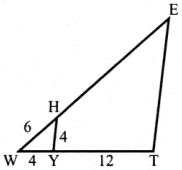Statements Reasons 1. ∠EWT = ∠HWY Common angle 2.  ∠ETW = ∠HYW Since YH || TE, corresponding angles 3. ∠WET = ∠WHY Since YH || TE corresponding angles 4. △WHY ~ △WET By AAA criteria

Also △ WHY ~ △WET
∴ Corresponding sides are proportionated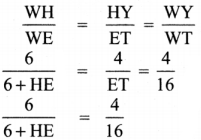⇒ 6+HE = $$\frac{6}{4}$$ × 16
⇒ 6 + HE = 24
∴ HE = 24 – 6
HE = 18
Again $$\frac{4}{\mathrm{ET}}=\frac{4}{16}$$
ET = $$\frac{4}{4}$$
ET = 16Question 9.
In the given figure, if △EAT ~ △BUN, find the measure of all angles.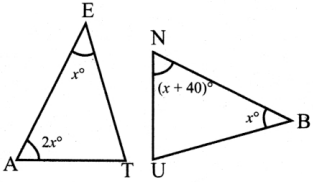Given △EAT ≡ △BUN
∴ Corresponding angles are equal
∴ ∠E = ∠B …… (1)
∠A = ∠U …… (2)
∠T = ∠N ……. (3)
∠E = x°
∠A = 2x°
Sum of three angles of a triangle = 180°
In △ EAT, x + 2x + ∠T = 180°
∠T = 180° – (x° + 2x°)
∠T = 180° – 3x°
Also in △BUN
(x + 40)° + x° + ∠U = 180°
x + 40° + x + ∠U = 180°
2x° + 40° + ∠U = 180°
∠U = 180° – 2x – 40° = 140° – 2x°
Now by (2)
∠A = ∠U
2x = 140° – 2x°
2x + 2x = 140°
4x = 140°
x = $$\frac{140}{4}$$ = 35°
∠A = 2x° = 2 × 35° = 70°
∠N = x + 40° = 35° + 40° = 75°
∴ ∠T = ∠N = 75°
∠E = ∠8 = 35°
∠A = ∠U = 70°Question 10.
In the given figure, UB || AT and CU ≡ CB Prove that △CUB ~ △CAT and hence △CAT is isosceles.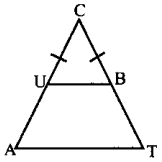Statements Reasons 1. ∠CUB = ∠CBU ∵ In △CUB, CU = CB 2. ∠CUB = ∠CAB ∵ UB || AT, Corresponding angle if CA is the transversal. 3. ∠CBU = ∠CTA CT is transversal UB || AT, Corresponding angle commom angle. 4. ∠UCB = ∠ACT Common angle 5. △CUB ~ △CAT By AAA criteria 6. CA = CT ∵ ∠CAT = ∠CTA 7. Also △CAT  is isoceles By 1, 2 and 3 and sides opposite to equal angles are equal.Objective Type Questions

Question 11.
Two similar triangles will always have _______ angles
(A) acute
(B) obtuse
(C) right
(D) matching
(D) matching

Question 12.
If in triangles PQR and XYZ, $$\frac{\mathrm{PQ}}{\mathrm{XY}}=\frac{\mathrm{QR}}{\mathrm{ZX}}$$ then they will be similar if
(A) ∠Q = ∠Y
(B) ∠P = ∠X
(C) ∠Q = ∠X
(D) ∠P ≡∠Z
Ans:
(C) ∠Q = ∠XQuestion 13.
A flag pole 15 m high casts a shadow of 3m at 10 a.m. The shadow cast by a building at the same time is 18.6m. The height of the building is
(A) 90 m
(B) 91 m
(C) 92 m
(D) 93 m
(D) 93 m

Question 14.
If ∆ABC – ∆PQR in which ∠A = 53° and ∠Q = 77°, then ∠R is
(A) 50°
(B) 60°
(C) 70°
(D) 80°